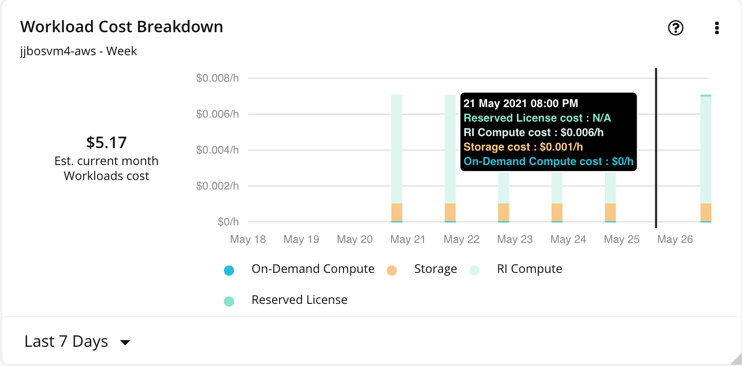# Entity Cost Data

The JSON for an entity can include cost data. This section describes the cost data as it relates to an entity.

## Sample Cost Data

This listing shows a sample entity object, with cost data included. Note that to make it easier to focus on cost, this listing omits some of the usual entity fields (for example, the `metric` field).

Click to Show/Hide:
```{
"timestamp" : "2021-05-21T19:59:33",
"entity": {
"oid": 73978235908269,
"name": "jjbosvm4-aws",
"state": "POWERED_ON",
"environment": "CLOUD",
"type": "VIRTUAL_MACHINE",
"cost": {
"unit": "\$/h",
"total": 0.0070848083,
"category": {
"ON_DEMAND_COMPUTE": {
"total": 0.0,
"source": {
"ON_DEMAND_RATE": 0.0126,
"RI_INVENTORY_DISCOUNT": -0.0126
}
},
"total": 0.0,
"source": {
"RI_INVENTORY_DISCOUNT": 0.0
}
},
"STORAGE": {
"total": 0.00078480854,
"source": {
"ON_DEMAND_RATE": 0.00078480854
}
},
"RI_COMPUTE": {
"total": 0.0063,
"source": {
"UNCLASSIFIED": 0.0063
}
}
}
},
"metric": {
...
},
"related": {
...
},
"attrs": {
...
}
}
}
```

## `entity.cost` Fields

The `cost` field contains the following data:

• `unit`: The unit of the cost value; for example, `\$/h`.
• `total`: The total cost for the given entity.
• `category`: A cost breakdown by category. One entity can include one or more of these categories:
• `ON_DEMAND_COMPUTE`
• `ON_DEMAND_LICENSE`
• `RESERVED_LICENSE`
• `STORAGE`
• `RI_COMPUTE`
• `IP`
• `SPOT`

Each category can include the following fields to give a total for that category, and to itemize the costs that contribute to that total:

• `total`: The total cost for given category.
• `source`: A breakdown of the total by source. The source can include the following types:
• `ON_DEMAND_RATE`
• `RI_INVENTORY_DISCOUNT`
• `BUY_RI_DISCOUNT`
• `UNCLASSIFIED`

Each source type gives the cost associated with that type. For example, the sample listing includes the following for on-demand compute costs:

``````"ON_DEMAND_COMPUTE": {
"total": 0.0,
"source": {
"ON_DEMAND_RATE": 0.0126,
"RI_INVENTORY_DISCOUNT": -0.0126
}
}
``````

This gives two sources for the category cost. The total is zero because the `ON_DEMAND_RATE` is canceled out by the `RI_INVENTORY_DISCOUNT`.

The cost in the sample listing matches this Workload Cost Breakdown chart:

• The total of \$5.17 is the product of `entity.cost.total` (which is \$0.0070848083 per hour) times the hours per month (730).
• The cost breakdown in the chart matches the the costs for the same categories; `ON_DEMAND_COMPUTE`, `STORAGE`, `RI_COMPUTE`, and `RESERVED_LICENSE`.The cost total and the breakdown by category give you the data you need to replicate the charts in Turbonomic. The breakdown by source also exposes how the costs are calculated, which is extra information that does not display in the user interface.